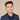# How To Get Substring Or Part Of A String In Swift

To get a substring or a part of a string in Swift you can use prefix and suffix.

## Using Prefix And Suffix

Here is an example if we wanted to get only the first 2 characters of a string using `prefix`.

``````let str = "ABCD"
print(str.prefix(2))
// AB``````

To get the last 2 characters of a string using `suffix`:

``````let str = "ABCD"
print(str.suffix(2))
// CD``````

## Get Part Of String Using Array Indexes

What if you want to get a part of a string that’s in the middle?

You can use the array index notation to do this on a string:

``````let str = "ABCD"
let start = str.index(str.startIndex, offsetBy: 1)
let end = str.index(str.startIndex, offsetBy: 2)
let range = start...end

let newString = String(str[range])

print(newString)
// BC``````

## Get Only One Character Of String

To get one character of the string you can convert the string into an array:

``````let str = "ABCD"
var someChar = Array(str)
print(firstChar)``````

This workaround is valid for most cases when you have a UTF8 or ASCII encoded string.

You can also use the index method provided above.

## Check If Contains Substring

Use the `contains` method to check if a substring exists:

``````import Foundation
let str = "ABCD"
print(str.contains("CD"))``````

Remember to `import Foundation` or else this won’t work!

If you liked this post and want to learn more, check out The Complete iOS Developer Bootcamp. Speed up your learning curve - hundreds of students have already joined. Thanks for reading!## Eddy Chung

I teach iOS development on ZeroToAppStore.com.